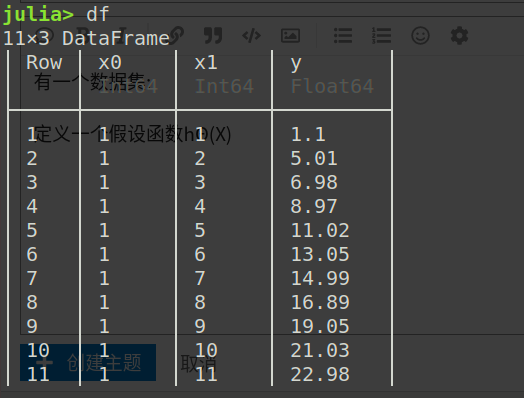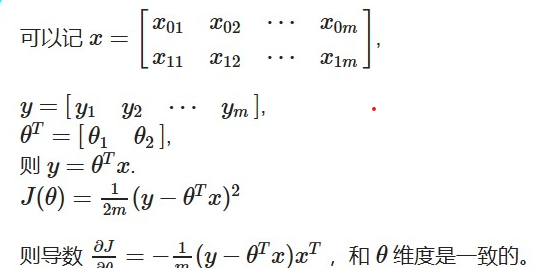# 请问这个代价函数的导数怎么求

#1Θ为矩阵[Θ01] (也是竖着的)

ps:我在网上复制的矩阵的markdown命令怎么在这里显示不出来？？

|x0 x11 x21 . . .xn1|
|x0 x12 x22 . . .xn2|
|. . . . . . . . . . . . . . |
|x0 x1m x2m . . . xnm|

0|
1|
|…|
n|

#2

J' = \frac{m}{2}\sum_i(\frac{\partial J}{\partial X_i}) = m\sum_i[X_i(\Theta^TX_i - Y_i)]

#3

y= \begin{bmatrix} y_{1} &y_{2}&\cdots& y_{m} \end{bmatrix},
\theta ^T= \begin{bmatrix} \theta_{1} &\theta_{2} \end{bmatrix},
y=\theta^T x.
J(\theta)=\frac{1}{2m}(y-\theta^T x)^2

x= \begin{bmatrix} x_{01} &x_{02}&\cdots&x_{0m}\\ x_{11} &x_{12}&\cdots&x_{1m} \end{bmatrix}
y= \begin{bmatrix} y_{1} &y_{2}&\cdots& y_{m} \end{bmatrix}
\theta ^T= \begin{bmatrix} \theta_{1} &\theta_{2} \end{bmatrix}

y=\theta^T x .
J(\theta)=\frac{1}{2m}(y-\theta^T x)^2 则导数 \frac{\partial{J}}{\partial{\theta}}= -\frac{1}{m}(y-\theta^T x)x^T ，和 \theta 维度是一致的。

#4

#5